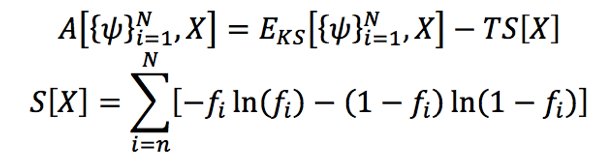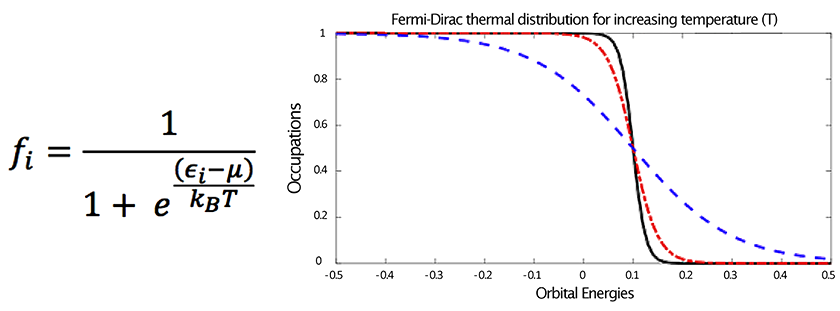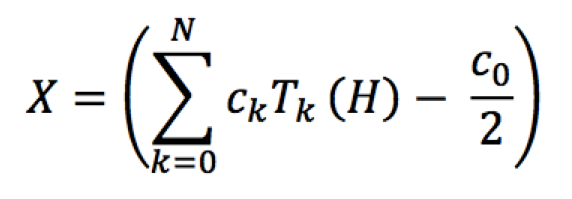# Algorithm for First-Principles Molecular Dynamics of Metals at Extreme Scales

### Daniel Osei-Kuffuor (15-ERD-032)

#### Abstract

First-principles molecular dynamics is an atomistic modeling technique for computing the electronic structure of a molecular system. The model includes electrons at the quantum level, which enables the creation of very realistic models of matter and atomic bonds. However, computational modeling of applications in material science using first-principles molecular dynamics is currently limited to systems with only a few atoms. This is primarily because the numerical solution of the model has cubic complexity. This project sought to develop, implement, and validate a truly scalable algorithm for first-principles molecular dynamics simulations of metals so that one can simulate a number of atoms directly proportional to the number of processors and take full advantage of the millions of processors available on Lawrence Livermore National Laboratory's BlueGene/Q supercomputer.

#### Background and Research Objectives

Low concentrations of an alloying species can dramatically change the physical properties of alloyed materials; however, modeling low concentrations requires a large total number of atoms and large-size simulations. Furthermore, modeling large defects, such as realistic dislocations produced by radiation damage, requires large atomistic simulations. Currently, realistic modeling of such applications is often out of reach for first-principles molecular dynamics because of the length of their time scale (Aarons et al. 2016; Osei-Kuffuor et al. 2014a and 2014b).

Our research objective was to develop a publicly available, parallel O(N) first-principles molecular dynamics dataset-scaling code that can handle systems with metallic properties. The work focused on extending a recently developed algorithm, whose performance grows linearly and in direct proportion to the number of atoms being simulated (Osei-Kuffuor et al. 2014a & 2014b), to metallic systems by (1) building a general reduced-basis set for computing the electronic structure of the model; (2) replacing the scalable calculation of selected elements of the inverse of an algebraic Gram matrix (M) with local computation of selected elements of a single-particle density matrix (X); and (3) developing and implementing robust solvers for the electron-structure problem. A new algorithm was developed to compute the single-particle density matrix in the reduced-basis set composed of non-orthogonal localized wave functions (representing the molecular orbitals). Exploiting the sparsity of this matrix can lead to a truly scalable first-principles molecular-dynamics simulation technology, making it tractable to solve large-scale applications and provide new insights into various materials’ properties.

#### Scientific Approach and Accomplishments

In dealing with metallic systems, two key challenges had to be addressed. The first challenge is scalability and parallel performance. Our strategy for dealing with scalability issues is based on building upon previous work with scalable algorithms for non-metallic systems. We leveraged algorithms that utilize short-range or nearest-neighbor communications, and built upon them to improve parallel scalability. One observed scaling bottleneck was in data access to assemble and perform key linear algebra operations. To alleviate this bottleneck, we implemented a new algorithm using hash tables (i.e., a compact dictionary-like data structure) to enable fast data access. The numerical solution scheme uses a domain-decomposition framework wherein the localized functions are distributed across the domains. In some cases, this distribution of functions can be unbalanced, resulting in poor performance. A new load-balancing algorithm, loosely based on the point-centered domain decomposition method, was developed to improve the distribution of workload in parallel and improve performance (Koradi 2000; Fattebert et al. 2016). To further improve computational efficiency and reduce the memory footprint of the molecular-dynamics algorithm, we implemented task-based mixed-precision arithmetic that utilizes single-precision data representation and arithmetic for appropriate linear-algebra kernels within the algorithm without compromising accuracy. A new memory-allocation strategy for distributed sparse matrices was also implemented.

The second challenge that needed to be addressed was the convergence of the numerical-solution scheme for the electron-structure calculations. The lack of a well-defined gap at the Fermi-level (i.e.,the top of the collection of electron energy levels at absolute zero temperature) for metallic systems makes it infeasible to employ traditional numerical strategies normally used for non-metallic systems. A common approach for the numerical solution of the discrete Fourier transform (DFT) equations for metallic systems is to solve a series of linear “surrogate” eigenvalue problems (by freezing the Hamiltonian operator or the energy eigenvalue), while enforcing a consistent single-particle density matrix. Typically, extrapolation strategies (such as the Anderson Acceleration) are employed to accelerate the solution of the “surrogate” problems. While this approach works in some cases, it has some weaknesses. In particular, the stopping criteria for the (inner) eigenvalue solutions is not well-defined. Furthermore, strong non-linearity in the problem invalidates the assumption of local quadratic behavior, making acceleration by extrapolation schemes infeasible. As a result, there is no guarantee of convergence using this approach, and several community codes, including O(N3) dataset-scaling codes, have convergence issues. A more robust strategy is to consider minimizing the Mermin functional, which is normally used in the study of metals, and which represents the Helmholtz free energy of the electron system (Fattebert et al. 2017; Dunn et al. 2017). For some finite temperature (T), this is given by Equation 1.Equation 1. Mermin functional representing the Helmholtz free energy of the molecular system. EKS represents the Kohn-Sham energy, TS is the entropic contribution, S is the entropy of the system, and ƒi = 1 ... N are the eigenvalues of X (also known as the "occupations").

At convergence, the occupations satisfy the Fermi-Dirac thermal-distribution function shown in Figure 1. This function describes the relationship between the Hamiltonian matrix (H), and the single-particle density matrix (X). At convergence of the optimization problem, H and X commute.Fermi-Dirac distribution function (left), where the i are the eigenvalues of the Hamiltonian matrix H (orbital energies), µ is the Fermi energy level, and kB is the Boltzmann constant. An illustration (right) of the Fermi-Dirac function for different values of kBT (black = 80, red = 40, blue = 10).

Marzari et al (1997) developed a two-step strategy for minimizing the Mermin functional based on decoupling the optimization problems of the wave functions and the occupations of these wave functions with respect to the energy of the system (Aarons et al. 2016). At every step of optimizing the wave functions, there is an inner process of optimizing the occupations keeping the set of wave functions fixed. This inner process can be represented as shown in Equation 2.Equation 2. Inner step of optimizing the occupations in the algorithm (Marzari et al. 1997), where λ is the line-minimization parameter and Xk is the result of diagonalization of the kth Hamiltonian matrix.

While this technique is quite effective, in some cases the mapping between occupations close to zero and the corresponding orbital energies can be challenging to resolve (ill-conditioning), which leads to convergence issues. Furthermore, computing the entropic contribution to the free-energy functional requires diagonalization (or some polynomial expansion approximation) of the (dense) X, which can be a scaling bottleneck. An alternate approach (Freysoldt et al. 2009) leads to a more stable algorithm by optimizing contributions of the molecular orbital energies (eigenvalues of H), instead of the occupations (eigenvalues of X). Thus, in the inner loop of the algorithm, X is replaced by H. We adopt this approach and adapt it to a non-orthogonal orbital formulation of the DFT equations. Furthermore, since H is sparse, this approach also enables us to develop a scalable strategy for computing the entropy using a Chebyshev approximation scheme defined on the orbital energies.

Each step of the iterative process to solve the minimization problem requires the computation of X to update H. A common approach is to compute X by diagonalization of H to compute the occupations. However, this approach has cubic scaling and clearly becomes a bottleneck for large systems. To efficiently compute the density matrix, we implemented a Chebyshev approximation strategy for general non-orthogonal orbitals. Using a Chebyshev polynomial expansion to approximate the Fermi-Dirac distribution function over the spectral bounds of H, X is computed as shown in Equation 3 (in simplified form without loss of generality).Equation 3. The Chebyshev expansion for computing the single-particle density matrix X, where ck are the Chebyshev coefficients and Tk are the Chebyshev interpolator nodes.

Computing the Chebyshev expansion requires several matrix multiplications that can make the calculation quite expensive. To improve efficiency, we draw from previous work and assemble a principal submatrix of H that adequately captures (in X) the long-range dynamics of the molecular orbitals centered in each subdomain. Using this approach, the Chebyshev expansion is performed locally, using a small principal submatrix with no communication across subdomains. In addition, since each principal submatrix is built with respect to only locally centered orbitals, only the corresponding columns of X need to be computed on each subdomain. This reduces the computations from matrix multiplications to sparse matrix-vector multiplications on each subdomain. In practice, the number of wave functions centered in each subdomain is three or less. The entries in X required on each subdomain are then obtained by gathering them from near neighbors using an efficient data distribution algorithm (Osei-Kuffuor et al 2014; Fattebert et al 2016). The result is a computationally efficient and scalable strategy for computing entries of the single-particle density matrix.

Computing the entropy for the entropic contribution to the free energy optimization is achieved in a similar way. The entropy function (S) is approximated by Chebyshev expansion, written concisely as in Equation 4.Equation 4. Concise form of the entropy (S) function, where p(X) is a polynomial expansion on X by a Chebyshev expansion, as in Equation 3.

Following the approach described above for computing X, we consider assembling a principal submatrix of X on each subdomain to perform the Chebyshev expansion. However, the computed columns of X are dense, resulting in a dense principal submatrix. To exploit sparsity and speed up computations, we reformulate the entropy as a function of the molecular orbital energies instead of the occupations. The result is a Chebyshev expansion of a function-of-a-function, where the argument is the sparse Hamiltonian matrix H, instead of X. The individual contributions to the trace summation of the entropy calculation are computed using a principal submatrix of H, constructed with respect to the centered wave functions on each subdomain. These partial sums are then summed together via an all-reduce operation to get the entropy.

In addition to these accomplishments, we have also implemented and validated new multiple-projector pseudopotentials. This will enable computational work with non-valence electrons and allow the code to be applicable to a wider class of problems.

#### Impact on Mission

The next-generation algorithms developed for this project will enable researchers to use first-principles molecular dynamics computations to calculate material properties by taking full advantage of today’s supercomputers with millions of cores. This supports the Laboratory’s core competency in high-performance computing, simulation, and data science. This work also addresses the need for better models of materials that can contribute to the Laboratory’s efforts in advanced materials and manufacturing.

#### Conclusion

Linear scaling algorithms are beginning to attract some attention in the materials science community. In addition, the increase in high-performance computing (HPC) capabilities encourages development of efficient algorithms that enable the study of the properties of materials using first-principles modeling. The goal of this project is to develop algorithms that enable efficient simulation of molecular systems with metallic properties, and support research of high interest to the Laboratory and DOE while utilizing Livermore’s HPC capabilities. Our work on this project has contributed to the development and implementation of scalable numerical strategies and robust algorithms that improve the performance of linear scaling algorithms for electron-structure calculations. Our recognition as ACM Gordon-Bell prize finalists at the 2016 Super Computing Conference demonstrates how contributions from this project can benefit science and maintain the Laboratory at the forefront of fast and accurate large-scale first-principles molecular-dynamics simulation capabilities.

The algorithms developed as part of this project have been implemented within the MGmol software. Thus far, we have evaluated the performance and robustness of our algorithms on small systems in order to enable validation against O(N3) results. The next step is to identify important applications of interest for MGmol to demonstrate its ability to simulate large metallic systems. We are also in the process of releasing MGmol to make it publicly available and promote collaborations with internal and external researchers. Our group has already received several collaboration requests from academia and research institutions.

#### References

Aarons, J., et al. 2016. “Perspective: Methods for Large-Scale Density Functional Calculations on Metallic Systems.” Journal of Chemical Physics 145, 220901. doi: 10.1063/1.4972007.

Fattebert, J. L., et al. 2016. “Modeling Dilute Solutions Using First-Principles Molecular Dynamics: Computing More Than a Million Atoms with Over a Million Cores.” SC '16: Proceedings of the International Conference for High Performance Computing, Networking, Storage and Analysis.

Koradi, R., 2000. “Point-Centered Domain Decomposition for Parallel Molecular Dynamics Simulation.” Computer Physics Communication 124 (2-3): 139—147. doi: 10.1016/S0010-4655(99)00436-1.

Marzari, N., et al. 1997. “Ensemble Density-Functional Theory for Ab Initio Molecular Dynamics of Metals and Finite-Temperature Insulators.” Physical Review Letters 79(7): 1337. doi:10.1103/PhysRevLett.79.1337.

Mermin, N. D., 1965. “Thermal Properties of the Inhomogenous Electron Gas.” Physics Review 137: A1441-A1443. doi: 10.1103/PhysRev.137.A1441.

Osei-Kuffuor, D., et al. 2014a. “A Scalable O(N) Algorithm for Large-Scale Parallel First-Principles Molecular Dynamics Simulations.” SIAM Journal on Scientific Computing 36(4): C353—375. doi: 10.1137/140956476.

——— 2014b. “Accurate and Scalable O(N) Algorithm for First-Principles Molecular-Dynamics Computations on Large Parallel Computers.” Physical Review Letters 112, 046401. doi: 10.1103/PhysRevLett.112.046401.

#### Publications and Presentations

Alexander, W. M., et al. 2015. “Approximating the Density Matrix for Electronics Structure Calculations.” LLNL-POST-675768.

Dunn, I. S., et al. 2017. “Fast, Scalable Ab Initio Molecular Dynamics.” LLNL-POST-735382.

Fattebert, J. L., et al. 2015. “Truly Scalable O(N) Algorithm for First-Principles Molecular-Dynamics Using Millions of Computer Cores.” LLNL-PRES-674473.

——— 2015a. “Truly Scalable O(N) Approach for First-Principles Molecular Dynamics (FPMD) of Non-Metallic Systems.” LLNL-PRES-668516.

——— 2015b. “O(N) Density Functional Theory Calculations: The Challenge of Going Sparse.” LLNL-PRES-678636.

——— 2016a. “Modeling Dilute Solutions Using First-Principles Molecular Dynamics: Computing More Than a Million Atoms with Over a Million Cores.” LLNL-PRES-708948.

——— 2016b. “Modeling Dilute Solutions Using First-Principles Molecular Dynamics: Computing More Than a Million Atoms with Over a Million Cores.” SC '16: Proceedings of the International Conference for High Performance Computing, Networking, Storage and Analysis, 12–22. doi: 10.1109/SC.2016.88. LLNL-CONF-689482.

——— 2017a. “O(N) Density Functional Theory Calculations: Beyond Ground State of Insulators.” LLNL-PRES-726750.

——— 2017b. “First-Principles Molecular Dynamics: Computing More than a Million Atoms with Over a Million Cores.” LLNL-POST-728652.

Osei-Kuffuor, D., et al. 2016. “A Parallel Algorithm for Approximating the Single Particle Density Matrix for O(N) Electronics Structure Calculations.” LLNL-PRES-688674.

&nbsp &nbsp